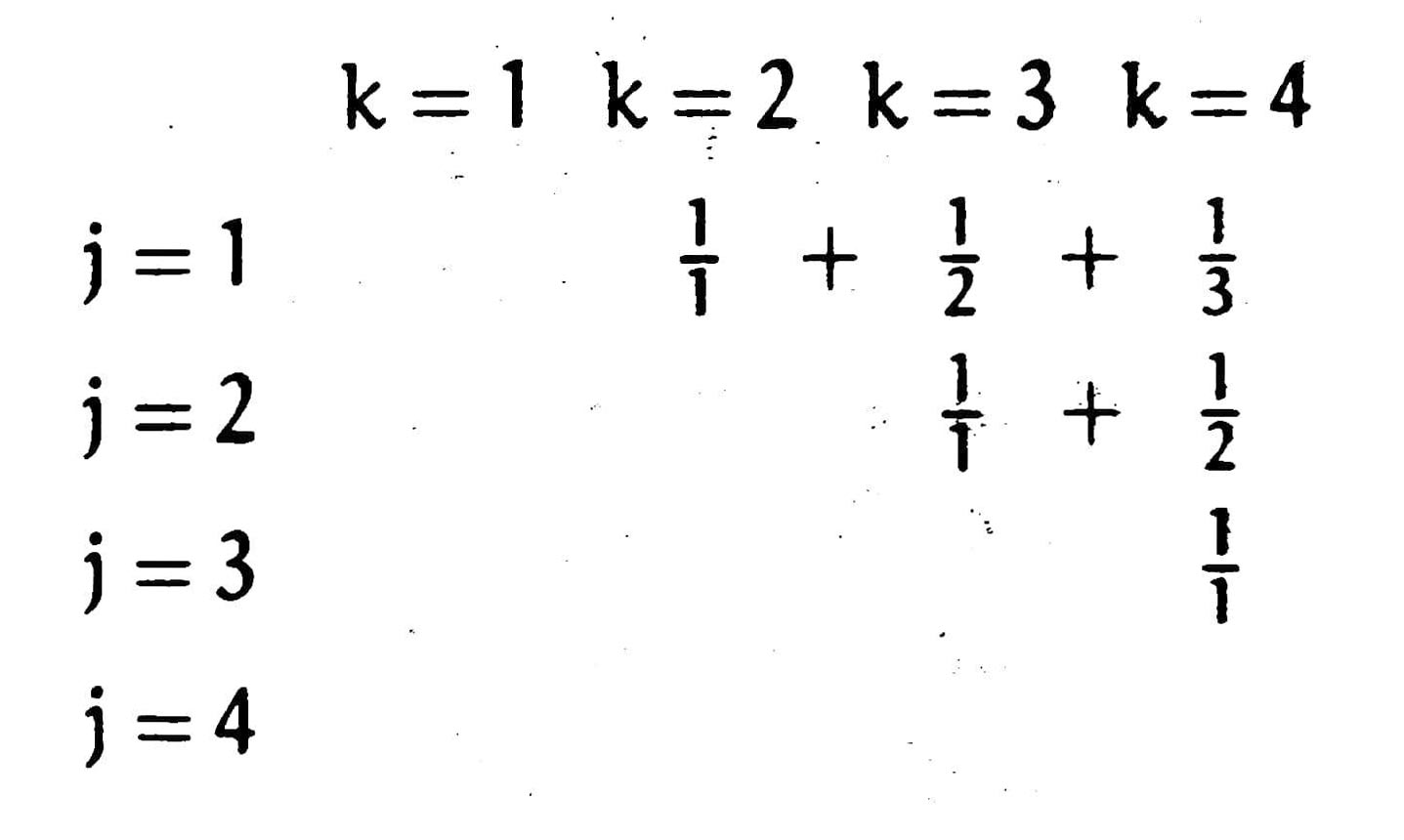# Chapter 2 Sums

## 2.1 Notation

The summation of an explicit sequence denoted as a succession of summations like $a_1+a_2+\cdots+a_n$ can be written in the delimited form $\sum_{k=1}^na_k$. Here, $a_k$ is called the summand, and $k$ is an index variable said to be bound to the summation. Summations can also be written in the general form like $\sum_{1\leq k\leq n}a_k$, making it easier for manipulations such as substitution of index variables.

## 2.2 Sums and Recurrences

There’s a neat relation between sums and recurrences. A sum like

$$S_n=\sum_{k=0}^na_k$$

is equivalent to the recurrence

\begin{aligned}S_0&=a_0;\\S_n&=S_{n-1}+a_n,\quad\text{for n>0.}\end{aligned}

Therefore we can evaluate a sum using methods of solving recurrences, such as the repertoire method we’ve learnt in Chapter 1.

We developed a technique that can reduce any recurrence of the form

$$a_nT_n=b_nT_{n-1}+c_n$$

to a sum, by multiplying both sides by a summation factor $s_n$, such that $s_nb_n=s_{b-1}a_{n-1}$. Then we can view $s_na_nT_n$ as a whole and go on.

## 2.3 Manipulation of Sums

To evaluate sums in closed form or to simplify sums, the key to success is the ability to change one summation into another under a few rules.

Let $K$ be any finite set of integers. Sums over the elements of $K$ can be transformed by using these three rules:

\begin{aligned}\sum_{k\in K}{ca_k}&=c\sum_{k\in K}{a_k};&&\text{(distributive law)}\\\sum_{k\in K}(a_k+b_k)&=\sum_{k\in K}a_k+\sum_{k\in K}b_k;&&\text{(associative law)}\\\sum_{k\in K}a_k&=\sum_{p(k)\in K}a_{p(k)}.&&\text{(commutative law)}\end{aligned}

The commutative law allows us to reorder terms. Here $p(k)$ is any permutation of the set of all integers. Actually, it’s a special case of a more generalized rule:

Suppose there’s an arbitrary function $f:J\to K$ that takes an integer $j\in J$ into an integer $k\in K$. The formula is

$$\sum_{j\in J}a_{f(j)}=\sum_{k\in K}a_k\#f^-(k),$$

where $\#f^-(k)$ stands for the number of elements in the set $f^-(k)=\big\{j\mid f(j)=k\big\}$.

If $f$ is an one-to-one correspondence between $J$ and $K$, we have $\#f^-(k)=1$ for all $k$, and the formula reduces to the commutative law.

## 2.4 Multiple Sums

\begin{aligned}\sum_{P(j,k)}a_{j,k}&=\sum_j\sum_ka_{j,k}\big[P(j,k)\big]=\sum_k\sum_ja_{j,k}\big[P(j,k)\big];&&\text{(interchanging the order of summation)}\\\sum_{\substack{j\in J\\k\in K}}a_jb_k&=\left(\sum_{j\in J}a_j\right)\left(\sum_{k\in K}b_k\right).&&\text{(general distributive law)}\end{aligned}

Another representation of the law of interchanging the order of summation is

$$\sum_{j\in J}\sum_{k\in K(j)}a_{j,k}=\sum_{k\in K^\prime}\sum_{j\in J^\prime(k)}a_{j,k}.$$

Here the sets $J$, $K(j)$, $K^\prime$ and $J^\prime(k)$ must be related in such a way that

$$[k\in K]\big[j\in J(k)\big]=[j\in J^\prime]\big[k\in K^\prime(j)\big].$$

It might be hard to understand at the first sight. But imagine that all items spread within a table like the one here:the left-hand side of the equation means for every $k$, sum up all items available in the $k$-th column, that is, all items in $J(k)$; the right-hand side is similar, $K^\prime(j)$ is the set of all items available in the $j$-th row.

## 2.5 General Methods

Method 0 is to look up. The authors suggested a few huge books for manual look ups. Fortunately, the on-line database OEIS is available; it is a more suitable tool than books for such knowledge.

Method 1 is guessing then proving. Since guessing needs flashes of inspiration, this method do not always work, and proving should in fact be a complement for other methods that establish sums from scratch.

Method 2 is the perturbation method. Perturb the sum a bit, then find relations between the perturbed sum and the original sum.

Method 3 is the repertoire method. This method still needs intuition to determine what form the recursion should be.

Method 4 uses infinite calculus to approximate the sum, then use other methods to compensate for the error terms.

Method 5 is to clever rewrite of the original sum. This method requires even more intuition than the repertoire method does. Expand a single sum to multiple sums to simplify the summand.

Method 6 is the topic of the next section.

Method 7 will be introduced in later chapters.

## 2.6 Finite and Infinite Calculus

Just like in calculus, we need a huge repertoire to evaluate sums effectively. Here are some useful formulas:

\begin{aligned}&x^{\underline{m+n}}=x^{\underline m}(x-m)^{\underline n};\\&\sum\nolimits_a^bc^x\delta x=\frac{c^b-c^a}{c-1};\\&\sum\nolimits_a^bx^{\underline m}\delta x=\begin{cases}\frac{x^{\underline{m+1}}}{m+1}\Big|_a^b,&\text{if m\neq-1;}\\H_x\Big|_a^b,&\text{if m=-1.}\end{cases}\end{aligned}

The relation of $\Delta(uv)=u\Delta v+{\rm E}v\Delta u$ yields the rule for summation by parts:

$$\sum u\Delta v=uv-\sum{\rm E}v\Delta u.$$

This rule is useful when $\sum{\rm E}v\Delta u$ is easier to evaluate than the original one.

## 2.7 Infinite Sums

In this section, we proved the validity of the three basic laws for absolutely convergent sums. Make sure the sum converges absolutely before applying these rules.